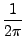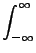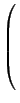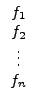## State Correlation Matrices

In the determination or optimization of the dynamic range of a filter the state-correlation matrices K and W are very important. K and W are defined as follows:

 K =SiFF*dω (32)

 W =SoG*Gdω (33)
in which F and G are a column and row matrix, respectively, defined as:

 F =(34)

 G = (g1, g2,…gn) (35)
and Si and So are the input and output weighting functions. The functions fi and gi are introduced in Section 10.2. From this section and the definitions given above it follows that

 | fi|22 = kii, (36)

 | gi|22 = wii. (37)
When this is compared to the dynamic range formulas in section 10.2 it is clear why K and W are so important for the evaluation of dynamic range. The optimization algorithm of Mullis and Roberts  and Hwang  use these matrices.

These matrices are automatically determined in FA whenever they are necessary. They can be written to the output by an output command, as in

	output: K W;

If this command is encountered and the matrices are still not determined in another analysis they are implicitly determined.

If Si = 1 and So = 1 , and the filter has not more than one pole on the imaginary axis, K and W are the unique solutions of the equations

 ATW + WA = - CTC (38) AK + KAT = - BBT (39)

and it is by solving these equations that FA determines the matrices if sol is set, which is default. This is certainly the fastest way known, and in most cases the most reliable one. In this way the noise (and other) integrals are determined without numerical integration.

If Si and/or So are not unity-valued constant functions K and W must be determined as a numerical integral, that is by directly evaluating (32) and (33). This can be done by setting the flag num (see section 9) before any (implicit) determination of the matrices. The effect of setting num is annihilated by setting sol, after which W and K are determined (again) by solving (38) and (39).

If the matrices are determined by numerical integration the relative or absolute accuracies by which the integrals are to be determined must be given. This is done via the keywords epsrel and epsabs, respectively, as follows.

epsrel = <value> ; epsabs = <value> ;
The default values for epsrel and epsabs are 1⋅10-6 . The numerical integration routine returns answers with an accuracy that corresponds to the largest of the two specified by epsrel and epsabs. epsrel is also used as a key to approximations that sometimes are necessary if singular matrices are encountered. If this is necessary FA always issues a warning.

Once the matrices are determined, they need not to be determined anymore. If by some transform command the filter is transformed, the K and W matrices are transformed accordingly. For that reason it is handy to determine these matrices before a lowpass-to-bandpass transformation, because after the transformation the order of the filter is twice the order it had, and the calculation will cost much more time.